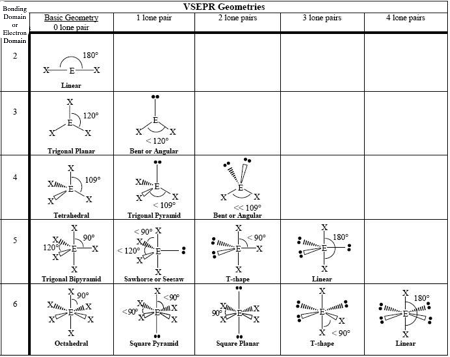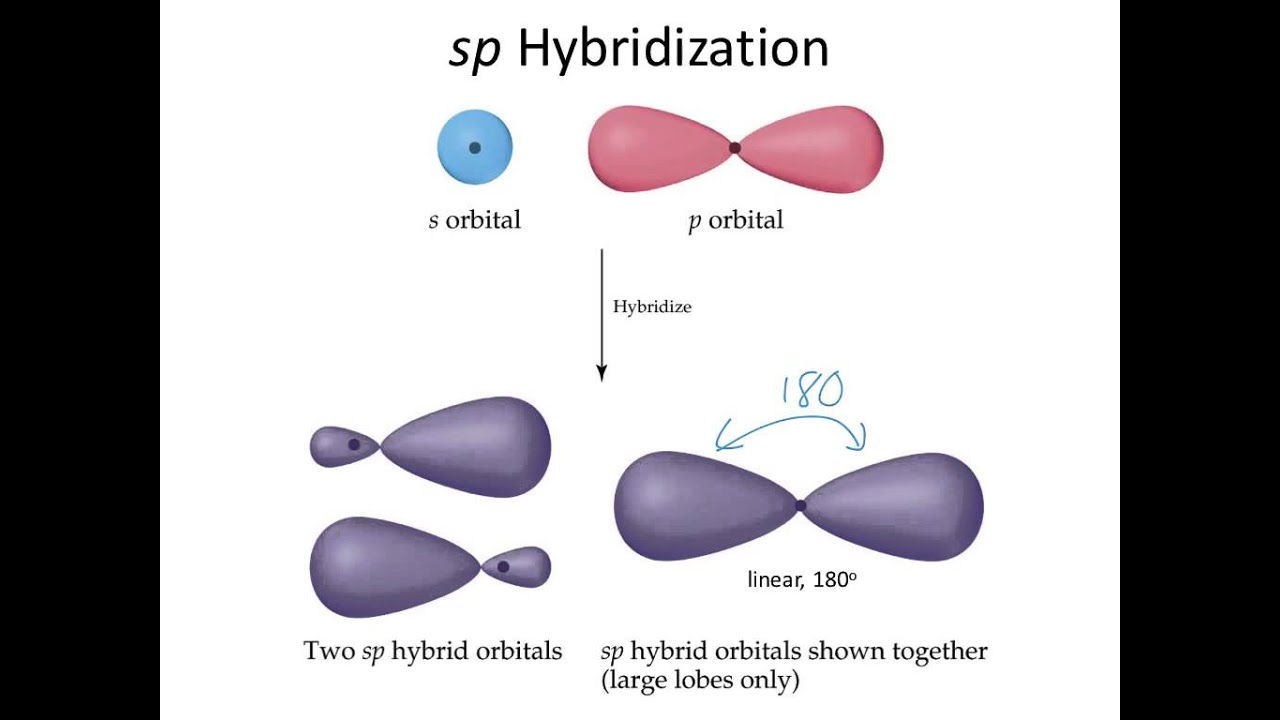# SeCl4 Lewis Structure, Geometry, Hybridization, and PolaritySelenium tetrachloride is an inorganic compound composed of one selenium atom and four chlorine atoms. Selenium belongs to group 16, and chlorine belongs to group 17.

It is a yellow-white volatile solid soluble in water.

In this article, we will understand the basic concepts required to predict Lewis structure, geometry, hybridization, and polarity of a given substance.

Contents

## Lewis Structure

Lewis structure is a 2D representation of the arrangement of atoms in a compound. It shows the arrangement of electrons around individual atoms.

According to Lewis, only valence electrons participate in bond formation, and hence only valence electrons are represented in Lewis symbols.

One compound can have more than one possible Lewis structure. We look for the structure that satisfies the octet rule and formal charges in such cases.

## Octet Rule

All the atoms of the main group elements prefer to have eight valence electrons (except period1 elements) to attain stability. This preference for eight electrons is called the octet rule.

Group 18 elements are considered most stable and have 8 valence electrons (except He). Thus, elements of other groups prefer 8 valence electrons to have noble gas-like stability.The octet rule is important for a stable Lewis structure, but many compounds can never follow the octet rule but are stable. Octet rule does not explain the stability of-

• Hypovalent compounds- central atom is electron-deficient, and its octet can never be completed. For example, AlCl3, etc.

• Hypervalent compounds- central atom is electron-rich due to the expansion of octets and has more than 8 valence electrons on the central atom. For example, SF6, etc.

• Odd electron species- some molecules have odd electrons and can never achieve octet as they cannot have an even number of electrons. For example, NO, etc.

## Formal Charge

It is a theoretical concept. It compares the electrons present on an isolated neutral atom with the electrons present on the atom in the compound. The formula for the formal Charge is

Formal Charge = (total number of valence shell electrons in an isolated atom) – (number of nonbonded electrons)- 0.5*(number of electrons involved in bond formation)

A negative formal charge is more stable on an electronegative atom than on an electropositive atom and vice-versa.

Steps for drawing Lewis structure for a given compound

1. First of all, count the number of electrons in the valence shell of each atom. Before drawing the Lewis structure, we need to know the number of valence shell electrons on all constituent atoms and their sum.

 Atom Atomic Number Group Number Valence electrons according to group number Electronic configuration (E.C.) Valence shell from E.C. Valence electrons from E.C. One Se 34 16 6 1s2 2s2 2p6 3s2 3p6 3d10 4s2 4p4 n=4 6 Four Cl 17 17 7 1s2 2s2 2p6 3s2 3p5 n=3 7 Total number of valence shell electrons= 6 + (7*2) = 20

2. Draw the lewis dot structure for elements.

We draw the Lewis structure of elements by arranging the valence shell electrons around the element’s chemical symbol.

The chemical symbols for Selenium and chlorine are Se and Cl, respectively. The Lewis dot structure for Se and Cl are as follows-3. Choose a suitable central atom for the compound.

We have to choose the central atom carefully. It must be the least electronegative atom out of the constituent atoms. Out of chlorine and Selenium, chlorine is more electronegative and cannot be the central atom

If the central atom is more electronegative than the side atoms, the central atom will keep the electron density towards itself.

Thus, Se is the central atom for this compound.

4. Draw a skeletal diagram.

In this step, we have to arrange the side atoms and central atoms suitably.5. Arrange the valence electrons around the elemental symbols.

The total valence shell electrons (calculated in step 1) are placed according to a predicted bond formation.6. Form bonds between adjacent atoms and try to complete the octet for all

Se has six valence electrons in the isolated state. It shares one electron with each chlorine. It can expand its octet due to the availability of vacant d orbitals. Thus, the octet rule is not satisfied for Se in this compound.

Each chlorine atom has 7 electrons in the valence shell in the free state, and it shares one electron with Selenium to have a fully filled configuration.7. Calculate the formal Charge on all atoms.

The net Charge on this compound is zero.

Atom Number of

electrons in the valence shell

according to the periodic table

Number of

electrons as

lone pair

(Number of

electrons

involved in

bonding )*0.5

Formal Charge

on atom

Se 6 2 8*0.5=4 6-2-4=0
Cl1 7 6 2*0.5=1 7-6-1=0
Cl2 7 6 2*0.5=1 7-6-1=0
Cl3 7 6 2*0.5=1 7-6-1=0
Cl4 7 6 2*0.5=1 7-6-1=0

Thus, the structure drawn in step 6 is the best Lewis structure for SeCl4.

## SeCl4 Geometry

Molecular geometry refers to the 3D arrangement of atoms of a molecule in space. VSEPR theory helps in determining the shape and geometry of a given compound.

VSEPR theory stands for valence shell electron pair repulsion theory.

This theory is based on the principle that the valence shell electrons of each atom in a molecule arrange themselves in such a way that the inter-electronic repulsion is minimum, and the arrangement becomes stable. The stable arrangement is called geometry.

Geometry and shape are often confused with each other. Geometry refers to the arrangement of bond pair of electrons, while shape refers to the arrangement of bond pair and lone pair of electrons.

### How To Predict SeCl4 Geometry Using VSEPR

1. First of all, we have to count the electrons in the valence shell of the central atom and let them be equal to A (arbitrary variable)

In the case of SeCl4, the central atom is Se. Se has 6 valence electrons. (Shown in step1 of drawing lewis structure)

A=6

2. Count the number of side atoms and let it be equal to B (arbitrary variable). In SeCl4, there are four side atoms and B=4

3. If the compound is charged, subtract the Charge from B for the positively charged compound and add the Charge to B for the negatively charged compound. This step can be avoided for neutral compounds.

In SeCl4, there is no contribution of Charge and B=4 only.

4. Add the contribution of side atoms and charge to the contribution of the central atom, i.e., A+B

For SeCl4, A+B=10

5. Divide A+B by 2 to find total electron pairs affecting the shape.

For SeCl4, there are 5 electron pairs.

6. Divide the total electron pairs as bonding and non-bonding. The bonding electron pair is equal to the number of side atoms.

For SeCl4, there are four side atoms. Thus, there are four bonding pairs of electrons and one non-bonding pair of electrons.

Using this information, one can easily find the geometry and shape for SeCl4 from the table below.

Electron geometry is trigonal pyramidal, and shape is a see-saw. The geometry and shape are different for compounds with lone pairs.## SeCl4 Hybridization

Hybridization is one of the fundamental concepts used to explain bond formation.

Hybridization involves mixing two or more atomic orbitals with comparative energy, size, and shape to form orbitals with the same energy, shape, and size.

The hybridized orbitals form bonds, and the extent of overlap is better than unhybridized orbitals.

For example, one 4s and one 4p can form two sp hybrid orbitals, but 4s and 8d cannot.In SeCl4, Se is the central atom. We are focusing on the hybridization of the central atom only.

In the ground state, Se has 2 unpaired electrons. It can only form two bonds. Promotion of electrons takes place from 4p to 4d, and 4 valence electrons become unpaired.### Steric Number Method

The steric number refers to the total number of electron pairs on the central atom.

For SeCl4, electron pairs=5 (shown while prediction of geometry using VSEPR)

Also, Steric number = number of (sigma bonds +lone pair on central atom)

Steric number for SeCl4= (4+1)=5

Hybridization comes out to be sp3d from the table.## SeCl4 Polarity

A compound is polar if it has a non-zero net dipole moment and non-polar if the net dipole moment is zero.

The exact value of net dipole moment can be found by vector addition of dipole moment of all the bonds in a molecule.

The dipole moment of a bond can be calculated using the formula

Dipole moment = charge* distance of separation between the charges

We need not calculate the exact value of dipole moment to determine polarity but can do a qualitative assessment of the compound for the same.

The dipole moment of a compound depends on

• Dipole moment, which in turn depends on the difference between the electronegativities of atom forming bonds.

• Geometry of compound

In SeCl4, only one type of bond is formed: ‘ Se-Cl’.

The electronegativity of Se and Cl is 2.55 and 3.16, respectively. The difference comes out to be 0.61. Thus, the bonds are polar.

Polar bonds do not guarantee a polar molecule. We consider the geometry and symmetry of the compound as well. The shape is a see-saw, and the bond vectors do not cancel each other out. Thus, the compound is polar.Related Topics

CH2Br2 Lewis Structure

CHF3 Lewis Structure

H2Se Lewis Structure

SeOCl2 Lewis Structure

SeF6 Lewis Structure

XeOF4 Lewis Structure

AlCl3 Lewis Structure

CH3CN Lewis Structure

SeF4 Lewis Structure

Cl2 Lewis Structure

AsH3 Lewis Structure

## Conclusion

Selenium tetrachloride is a covalent compound.

The Lewis structure drawn in the above sections is the most suitable. Se expands its octet and is a hyper-valent compound.

The geometry of the compound is trigonal pyramidal, and the shape is a see-saw. The hybridization of the central atom is sp3d.

It is a polar compound.